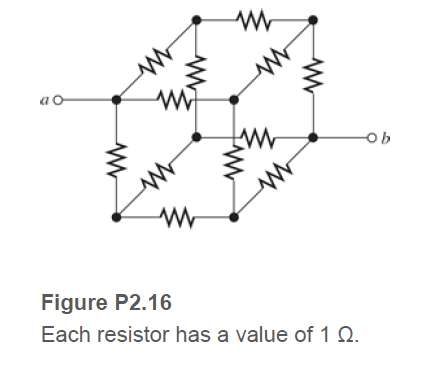# Figure P2.16 Each resistor has a value of 1 Q.

Question

Sometimes, we can use symmetry considerations to find the resistance of a circuit that
cannot be reduced by series or parallel combinations. A classic problem of this type is illustrated
in Figure P2.16. Twelve 1-Ω resistors are arranged on the edges of a cube, and terminals a and
b are connected to diagonally opposite corners of the cube. The problem is to find the resistance
between the terminals. Approach the problem this way: Assume that 1 A of current enters terminal a and exits through terminal b. Then, the voltage between terminals a and b is equal tothe unknown resistance. By symmetry considerations, we can find the current in each resistor.Then, using KVL, we can find the voltage between a and b.help_outlineImage TranscriptioncloseFigure P2.16 Each resistor has a value of 1 Q. fullscreen

### Want to see this answer and more?

Experts are waiting 24/7 to provide step-by-step solutions in as fast as 30 minutes!*

*Response times may vary by subject and question complexity. Median response time is 34 minutes for paid subscribers and may be longer for promotional offers.
Tagged in
Engineering
Electrical Engineering

### Circuit Theory